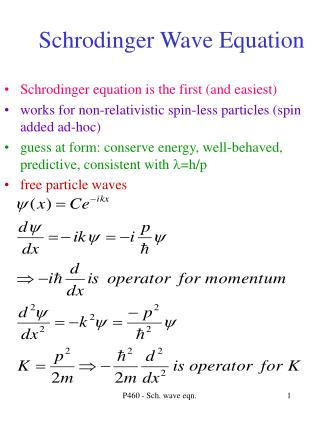DownloadDownload PresentationSchrodinger Wave Equation

# Schrodinger Wave Equation

Télécharger la présentation## Schrodinger Wave Equation

- - - - - - - - - - - - - - - - - - - - - - - - - - - E N D - - - - - - - - - - - - - - - - - - - - - - - - - - -
##### Presentation Transcript

1. Schrodinger Wave Equation • Schrodinger equation is the first (and easiest) • works for non-relativistic spin-less particles (spin added ad-hoc) • guess at form: conserve energy, well-behaved, predictive, consistent with l=h/p • free particle waves P460 - Sch. wave eqn.

2. Schrodinger Wave Equation • kinetic + potential = “total” energy K + U = E • with operator form for momentum and K gives Giving 1D time-dependent SE For 3D: P460 - Sch. wave eqn.

3. Operators • Operators transform one function to another. Some operators have eigenvalues and eigenfunctions Only some functions are eigenfunctions. Only some values are eigenvalues In x-space or t-space let p or E be represented by the operator whose eigenvalues are p or E P460 - Sch. wave eqn.

4. Interpret wave function as probability amplitude for being in interval dx P460 - Sch. wave eqn.

5. Example No forces. V=0 solve Schr. Eq Find average values P460 - Sch. wave eqn.

6. P460 - Sch. wave eqn.

7. Solving Schrodinger Equation • If V(x,t)=v(x) than can separate variables G is separation constant valid any x or t Gives 2 ordinary diff. Eqns. P460 - Sch. wave eqn.

8. G=E if 2 energy states, interference/oscillation 1D time independent Scrod. Eqn. Solve: know U(x) and boundary conditions want mathematically well-behaved. No discontinuities. Usually except if V=0 or y =0 in certain regions P460 - Sch. wave eqn.

9. Solutions to Schrod Eqn • Gives energy eigenvalues and eigenfunctions (wave functions). These are quantum states. • Linear combinationsof eigenfunctions are also solutions P460 - Sch. wave eqn.

10. Solutions to Schrod Eqn • Linear combinationsof eigenfunctions are also solutions. Asuume two energies • assume know wave function at t=0 • at later times the state can oscillate between the two states P460 - Sch. wave eqn.

11. The normalization of a wave function doesn’t change with time (unless decays). From Griffiths: Use S.E. to substitute for J(x,t) is the probability current. Tells rate at which probability is “flowing” past point x substitute into integral and evaluate The wave function must go to 0 at infinity and so this is equal 0 P460 - Sch. wave eqn.